### Home > PCT > Chapter Ch9 > Lesson 9.1.4 > Problem9-56

9-56.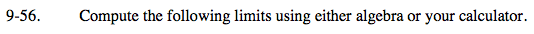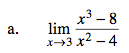Substitute 3 in for x.
You can do that because you do not have division by zero.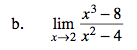Do NOT substitute 2 in for 'x' yet or you will have division by zero problem. Factor first, eliminating this problem. Then substitute 2 in for 'x'.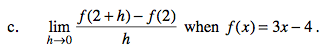$\lim_{x\rightarrow 0}\frac{(3(2+h)-4)-((3)(2)-4)}{h}$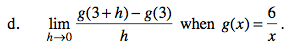$\lim_{x\rightarrow 0}\frac{\frac{6}{3+h}-\frac{6}{3}}{h}=\frac{\frac{18-6(3+h)}{3(3+h)}}{h}$